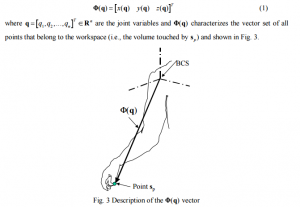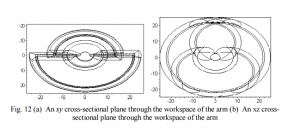# Cost Functions in Ergonomics

This is a guest post by Sarah Dantino

Cost functions are linear functions describing a relationship between an initiator and its results. They are often used in business and accounting to predict and minimize future costs.  A worked-out example of constructing and minimizing an average cost function is in the YouTube video below by James Sousa.

I was interested in looking into how these cost functions are used in the analytical approach to ergonomics, which is an applied science to configure the work space so that it is both safe and functional. In this mathematical approach, researchers use cost functions to model kinetics, which is the energy and motion of human limbs. Instead of measuring a financial cost, the results measure reachability, dexterity, stress, potential energy, etc.

The goal is to maximize reachability while minimizing stress at each joint. To do this, researchers determine the area of reach(boundary envelope) and move the work space to optimize these cost functions (Abdel-Malek, Yang, 2004). The points that we need within reach are called target points, whereas the contact point on the body (i.e. the tip of a finger) is called the specified point. Imagine a coordinate system originating at each joint and motion constraints depending on the magnitude and range of the vectors (limb portions). Similar coordinate systems on the human body are used in other areas of research such as physical therapy and robotics.

The end goal is to configure the work space where all the target points are inside the boundary envelope but the cost function is optimized; to do this, researchers create 2D and 3D graphs of these work space envelopes.It’s important to note that people are both physically and mentally affected by their environment. The productivity, efficiency, and safety of someone’s work can be maximized if careful decisions are made regarding the worker’s environment, and the analytic approach to ergonomics can help make these decisions.

Are there any workplace changes you have made over the years that have made it much more comfortable to work long hours at your desk? Share any ergonomic tips in the comments below!

References:

• http://www.merriam-webster.com/dictionary/ergonomics
• Abdel-Malek, K., Yang, J. (2004). A mathematical method for ergonomic-based design: Placement. International Journal of Industrial Ergonomics, November.
• K. Abdel-Malek, J. Yang, “A mathematical method for ergonomic-based design: Placement” International Journal of Industrial Ergonomics, November 2004.